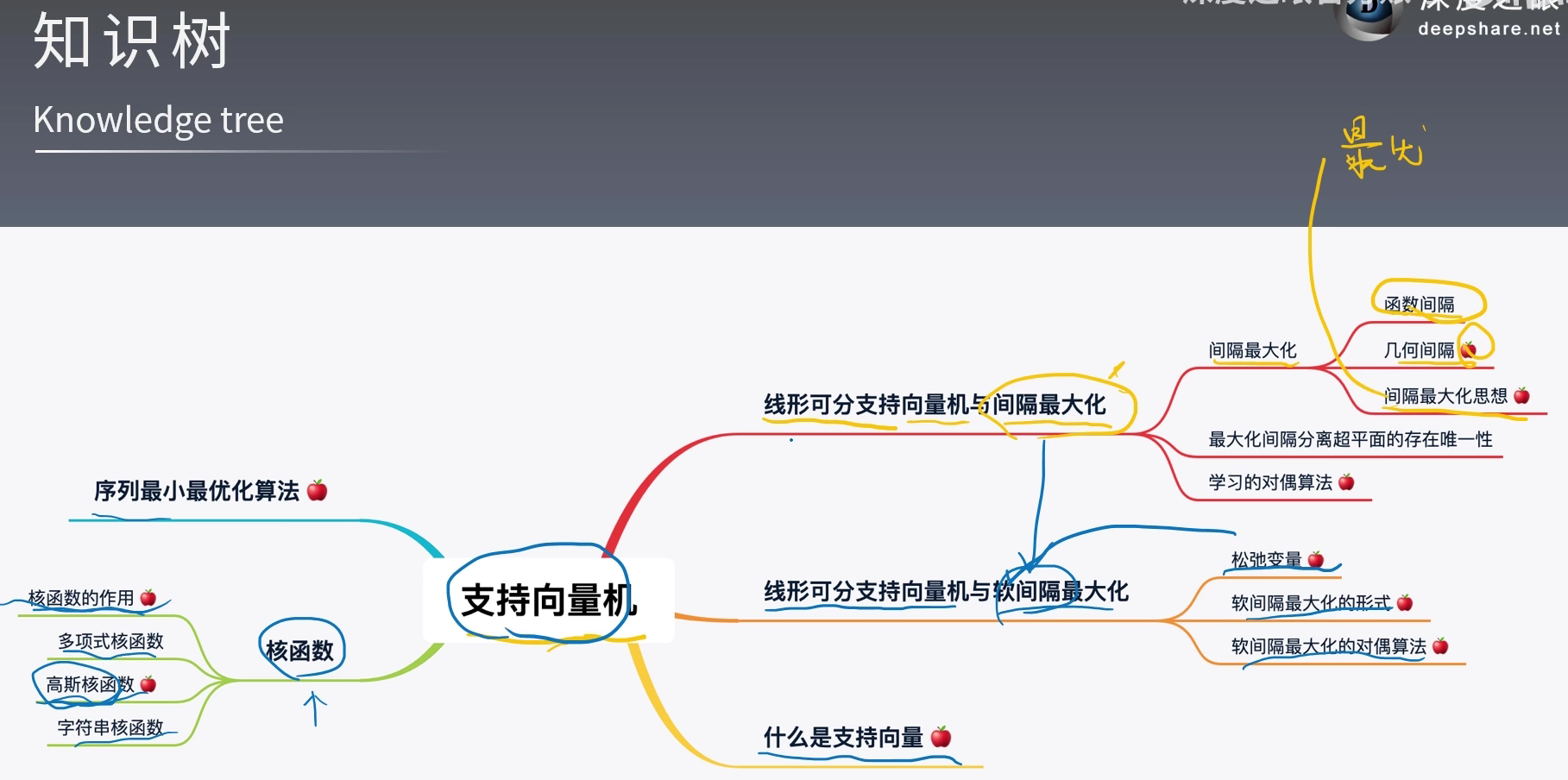# 我们想找到一个最好的、最优的超平面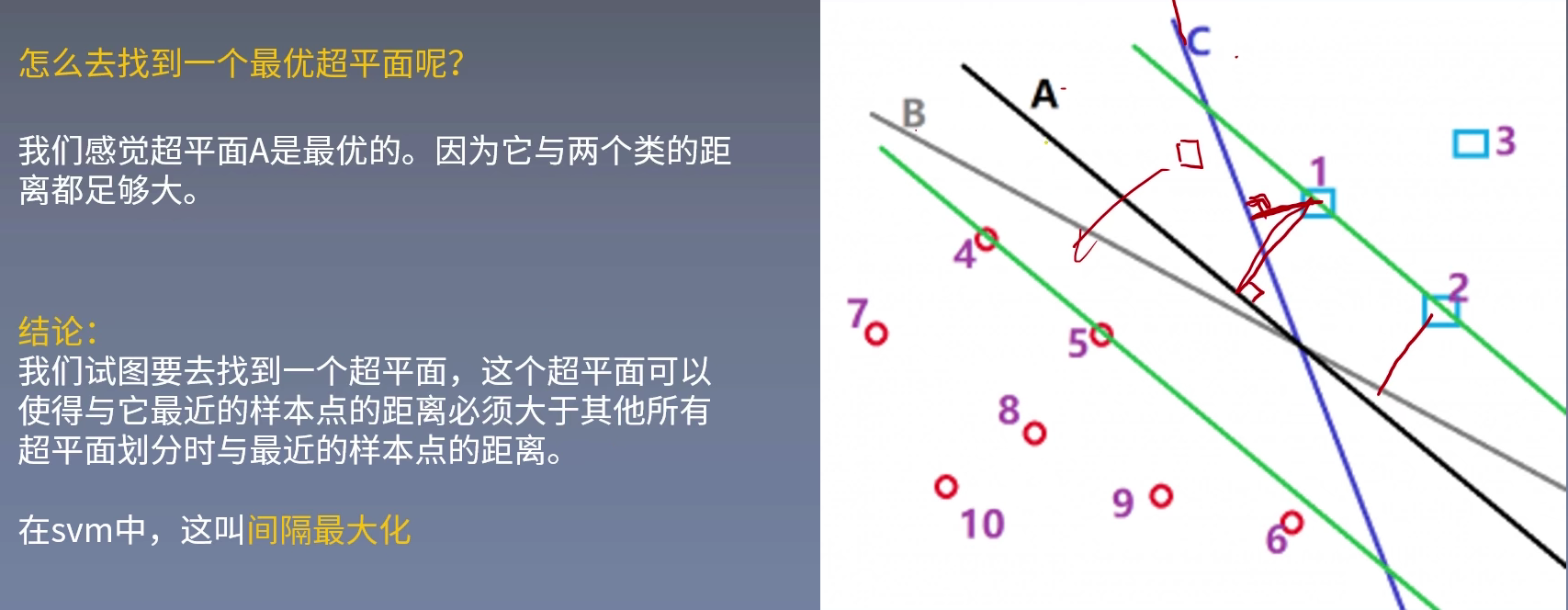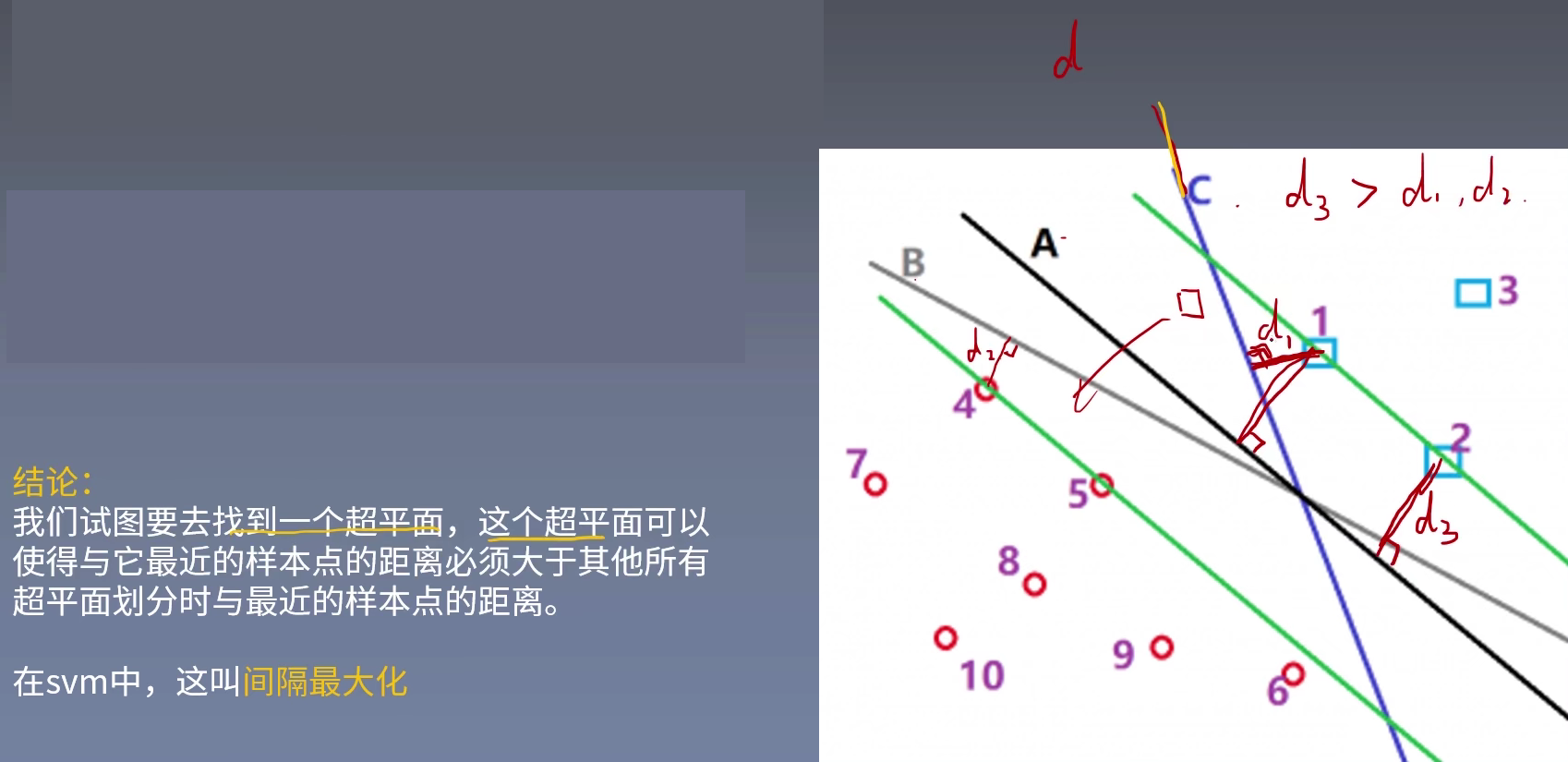## 低维不可分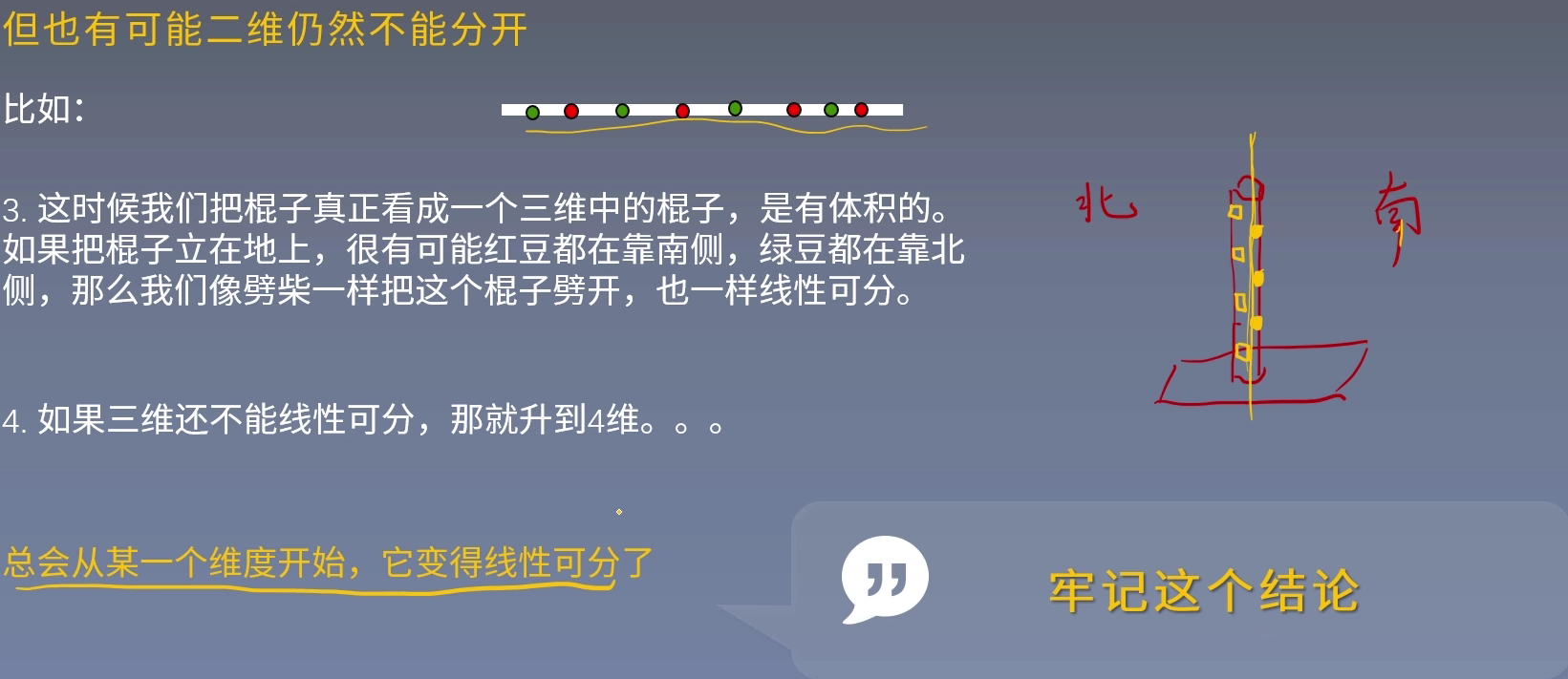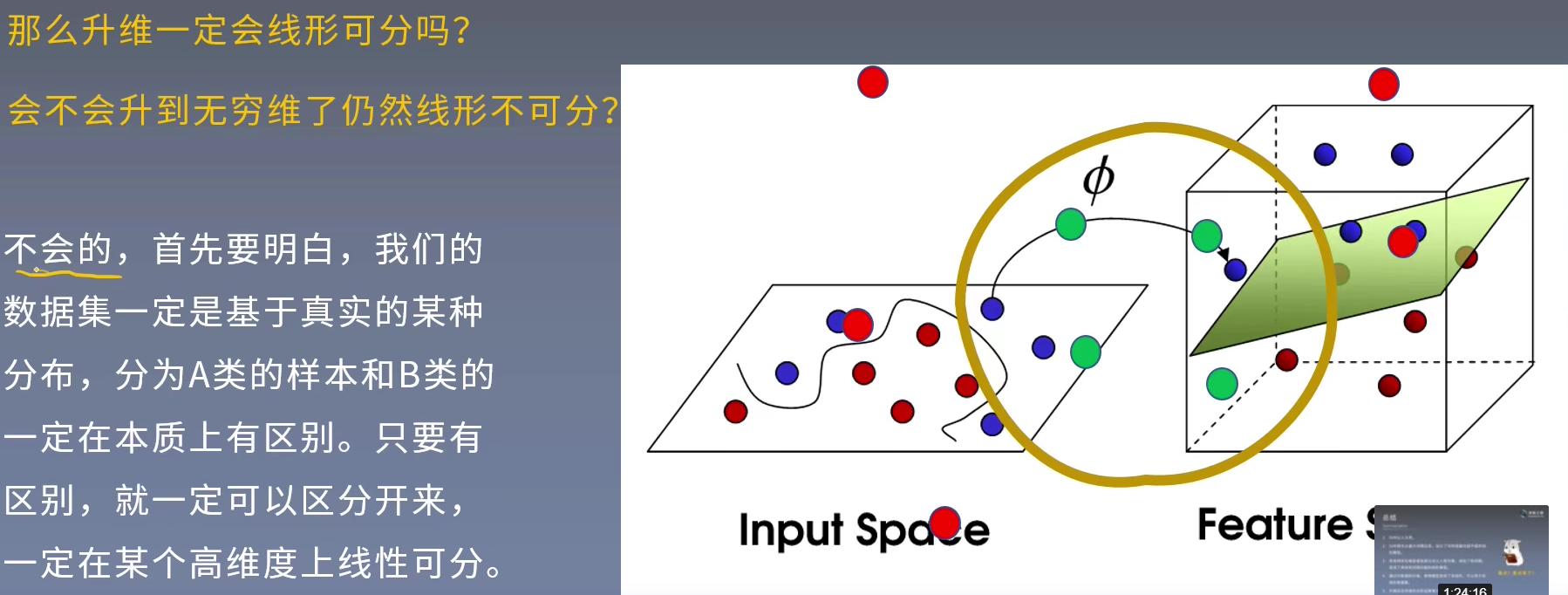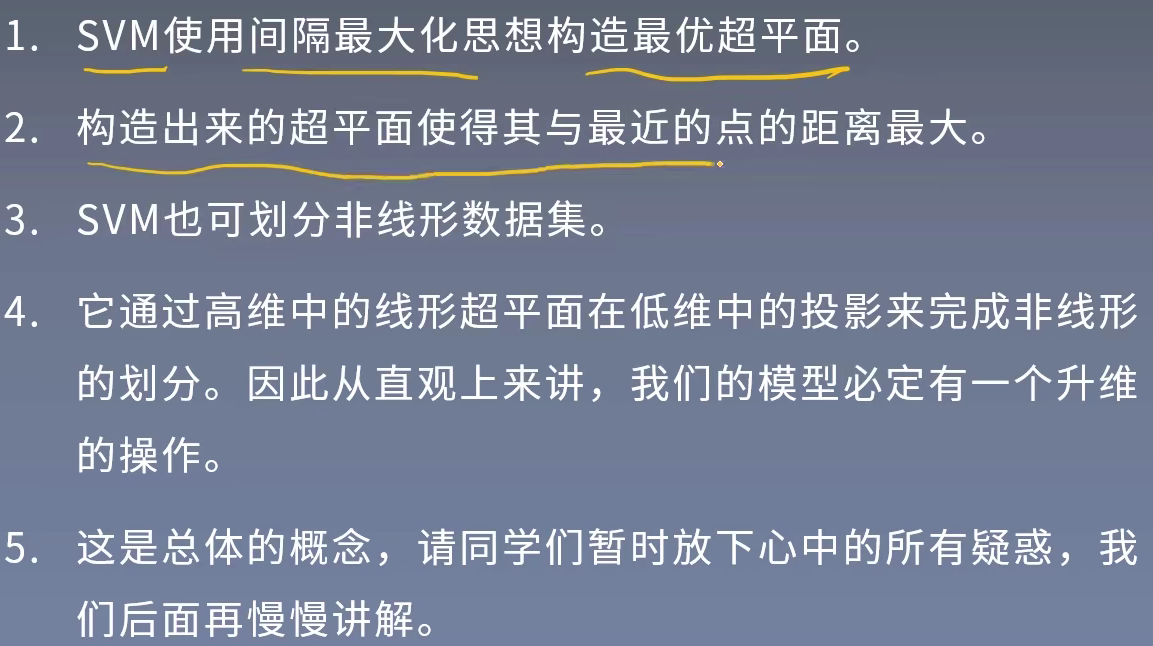# 支持向量机分类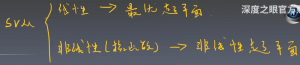# 函数间隔和几何间隔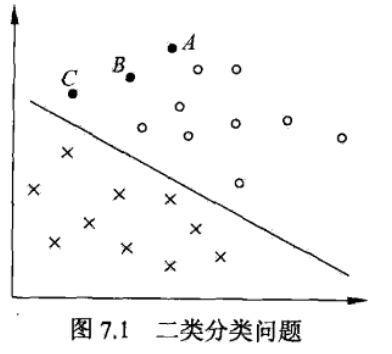$\hat{\gamma } = y_{i}(w^Tx+b)$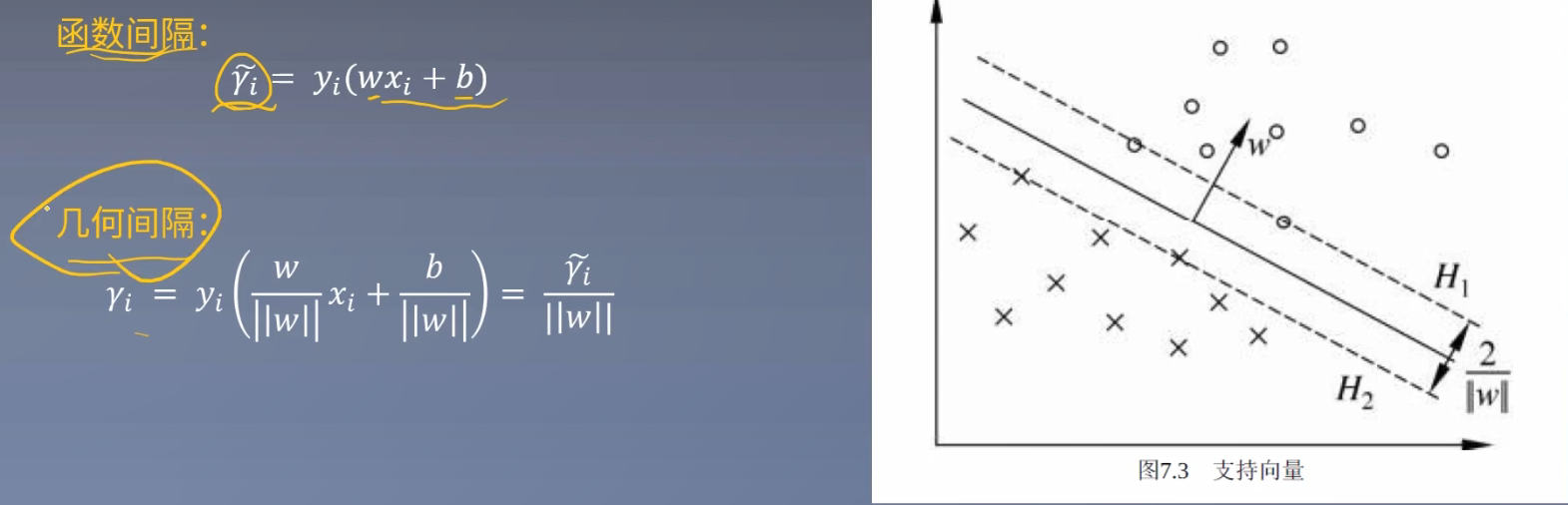https://www.bilibili.com/video/BV1g64y1h7Lc?p=17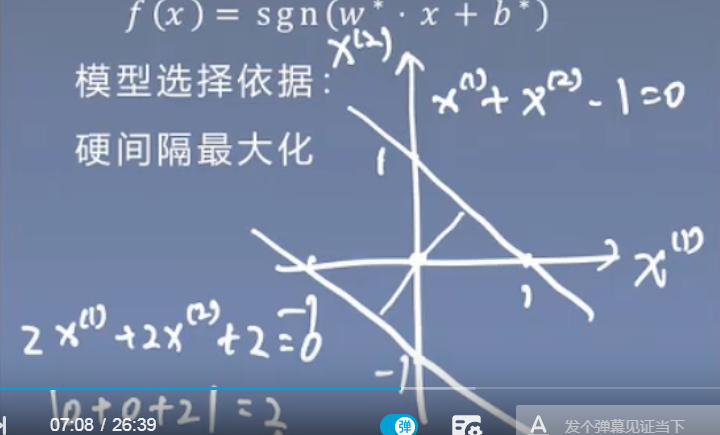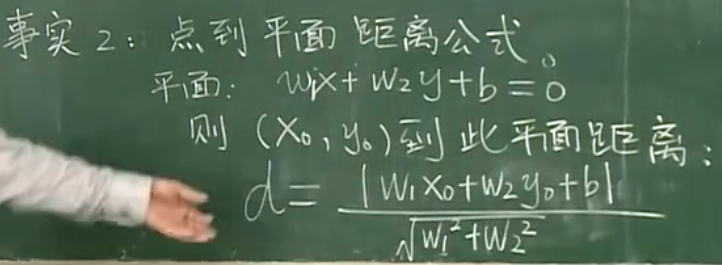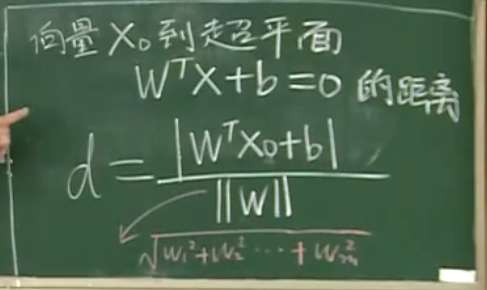# 间隔最大化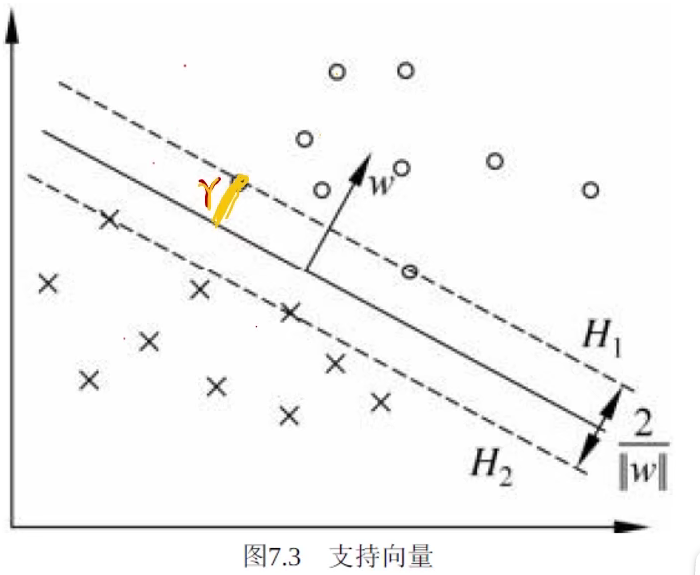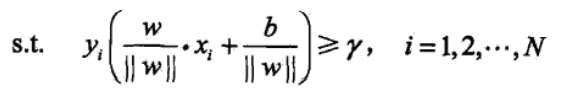## 用函数间隔得到等价最优化问题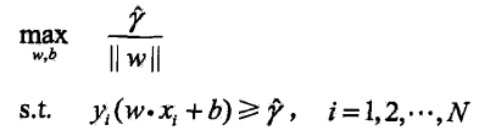## $\gamma$的取值不影响最优化的解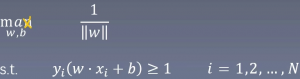## 为什么分子取1？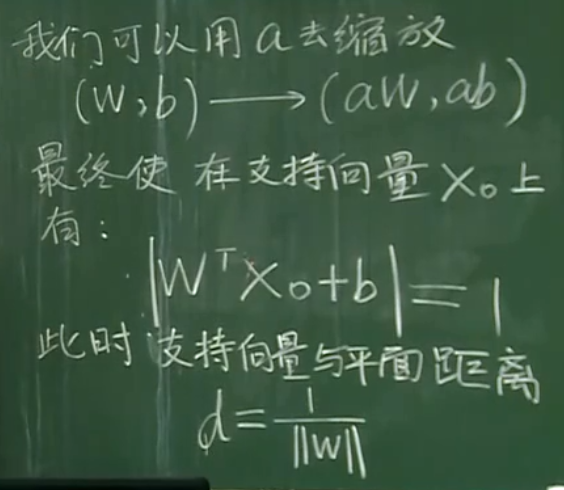a的作用就是将最适合的那个超平面移到中间的位置。

## 最大化转为最小化问题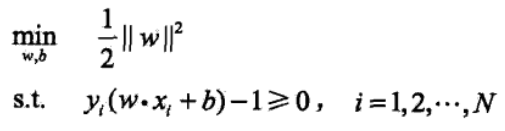## 拉格朗日乘子法

$L(w, b, \alpha ) = \frac{1}{2}||w|| ^2-\sum_{i=1}^{N}\alpha _{i}[y_{i}(w^Tx_{i}+b)+1]$

$=\frac{1}{2}||w|| ^2-\sum_{i=1}^{N}\alpha _{i}[y_{i}(w^Tx_{i}+b)]+\sum_{i=1}^{N}\alpha _{i}$

## 对偶问题

KKT条件为我们证明出来的对偶性质，它可以帮助我们推导。（我们暂时不用去看KTT推导，暂时认为是对的就可以了，知道就可以了！）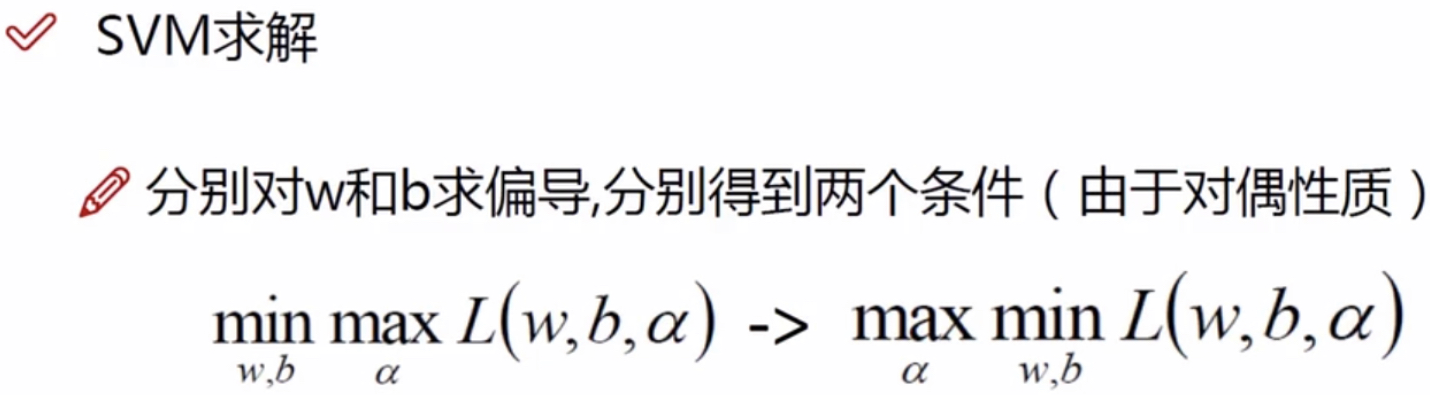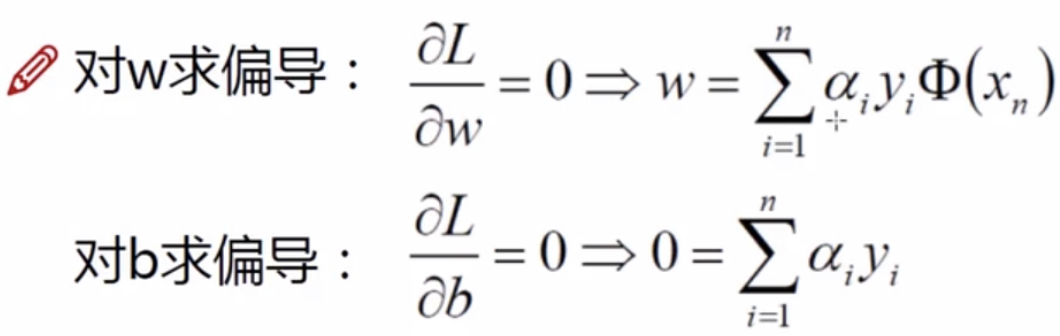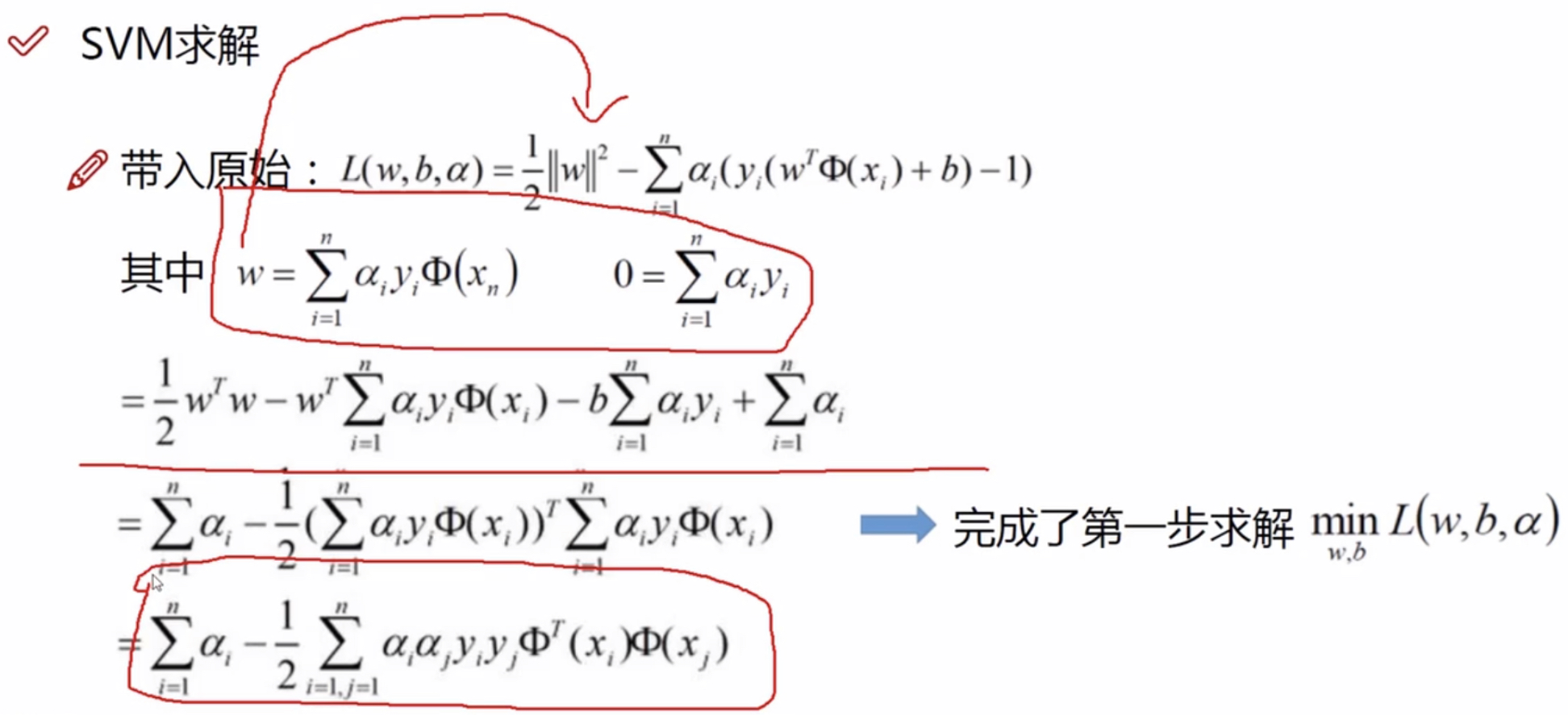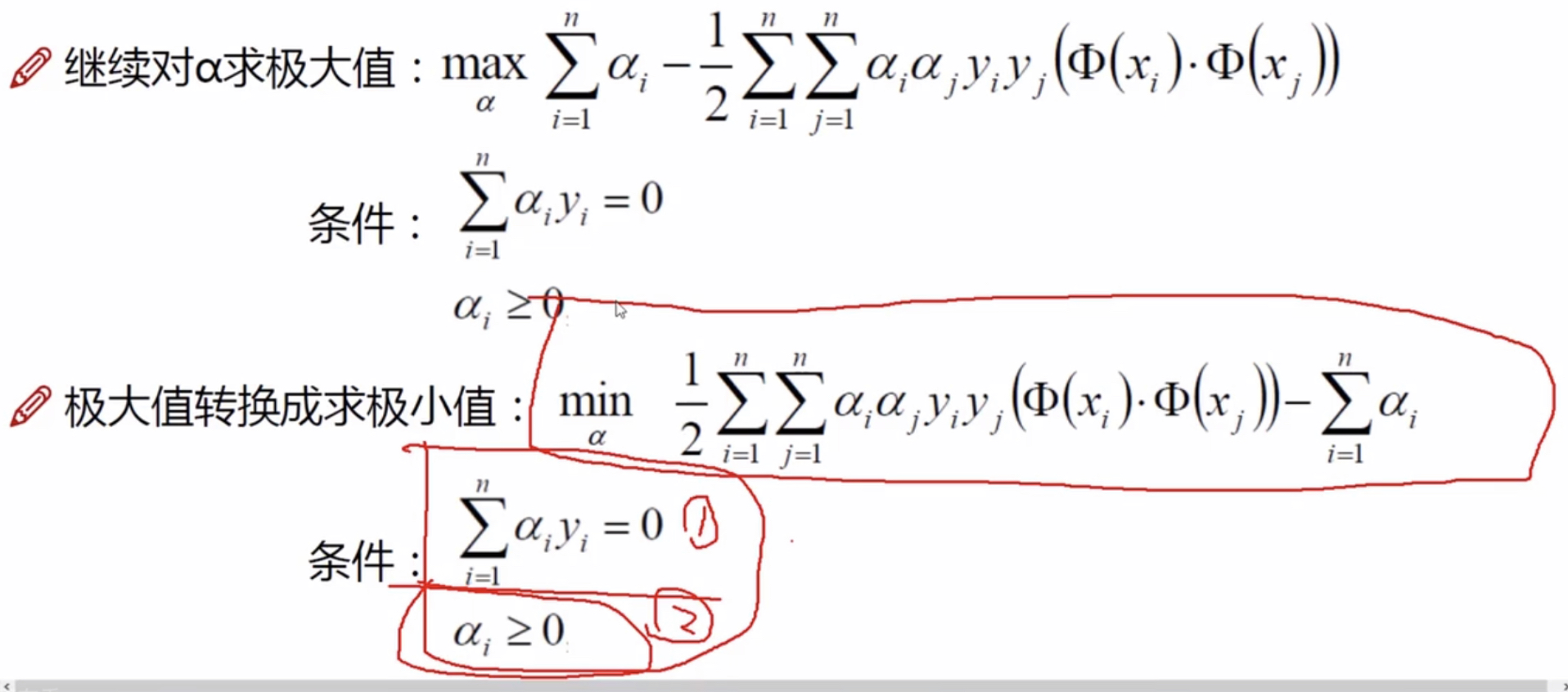# 支持向量机求解实例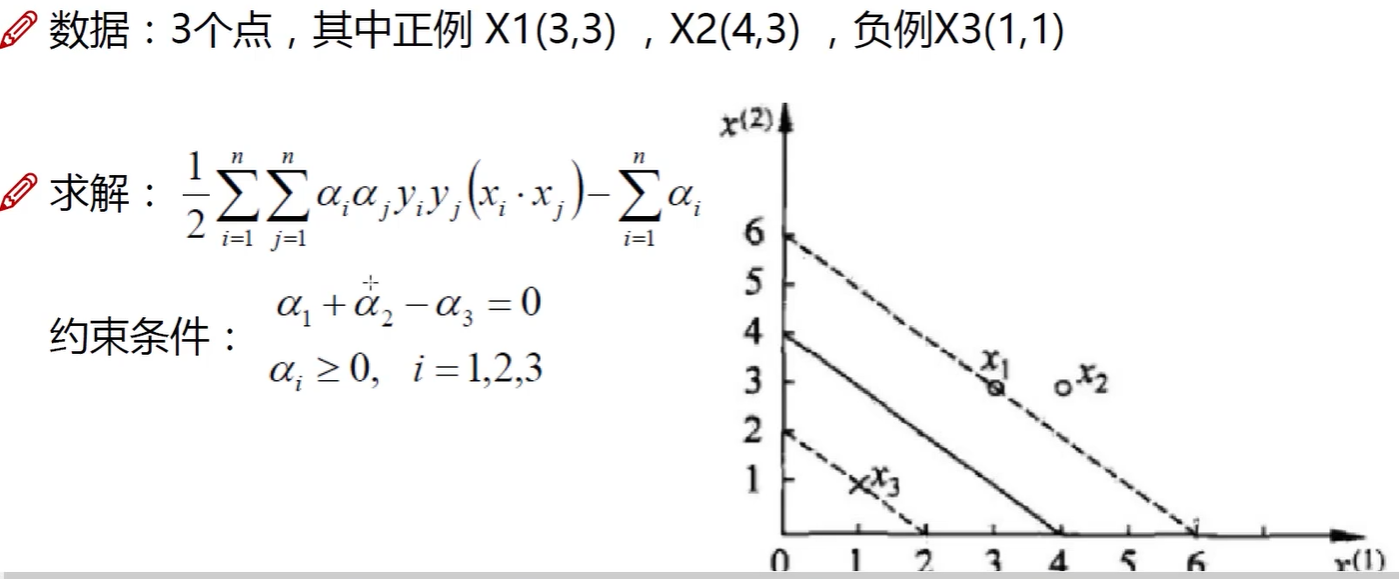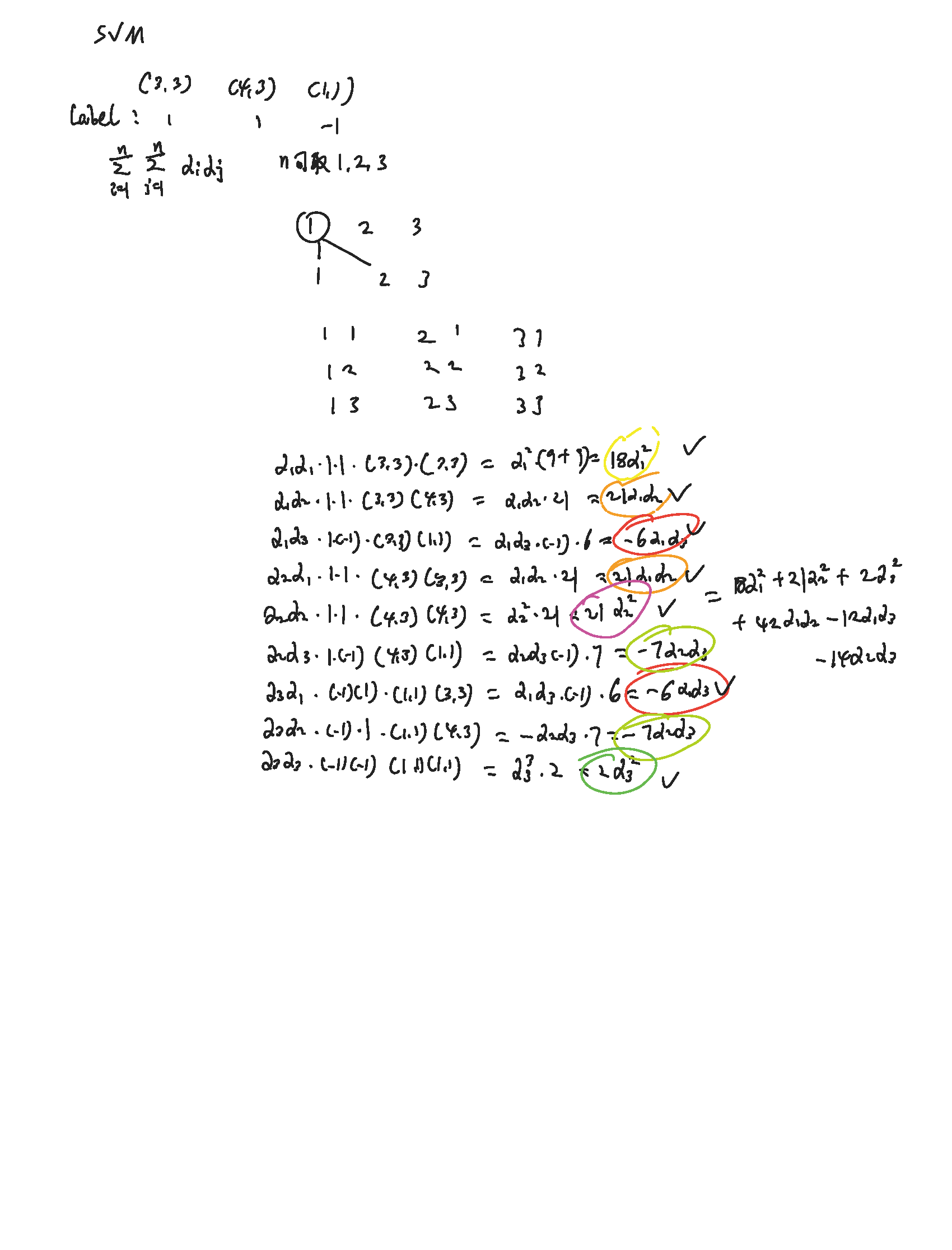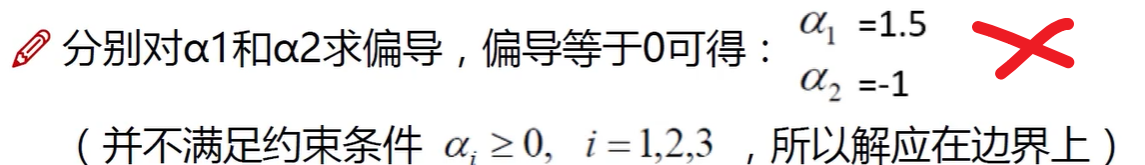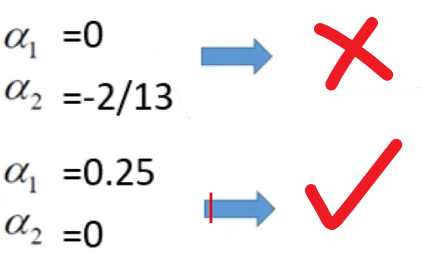## 哪些点是起作用的点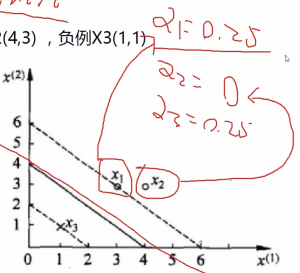https://www.bilibili.com/video/BV1i4411G7Xv?p=7

https://www.zhihu.com/question/38586401

https://www.zhihu.com/collection/740229096

https://b23.tv/BV1UR4y147AT/p8